# JavaScript：需要知道的部分

## 太多太多的選擇：switch case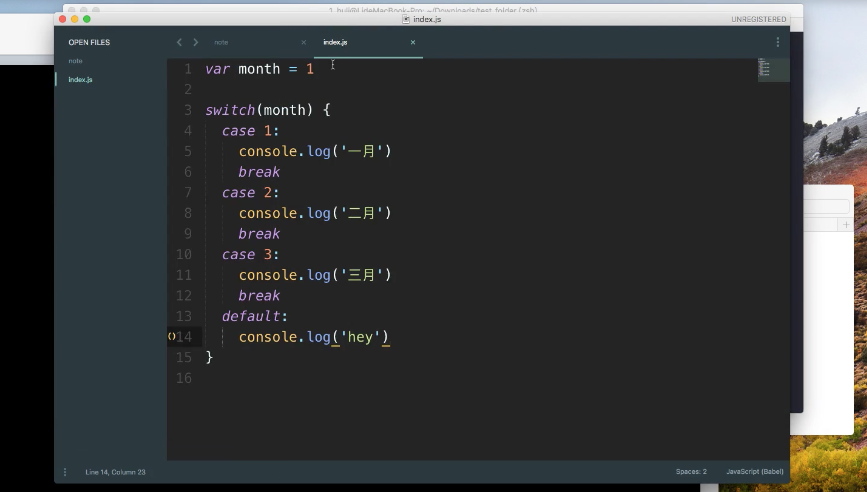var week = 1
switch(week){
case 1:
console.log("星期一")
brake
case 2:
console.log("星期二")
brake
defaule:
console.log("請輸入正確範圍")
}

var week = 1
switch(week){
case 1:
case 2:
console.log("星期一")
brake
defaule:
console.log("請輸入正確範圍")
}

case 可以接著寫，多條件指向同答案。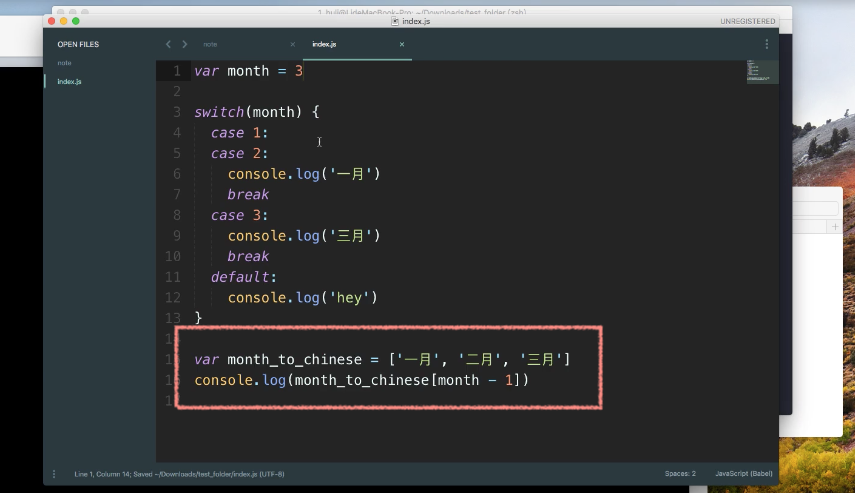## 一、二、三：三元運算子（Ternary）

if、else 判斷式中，可以簡化成三元運算子的形式。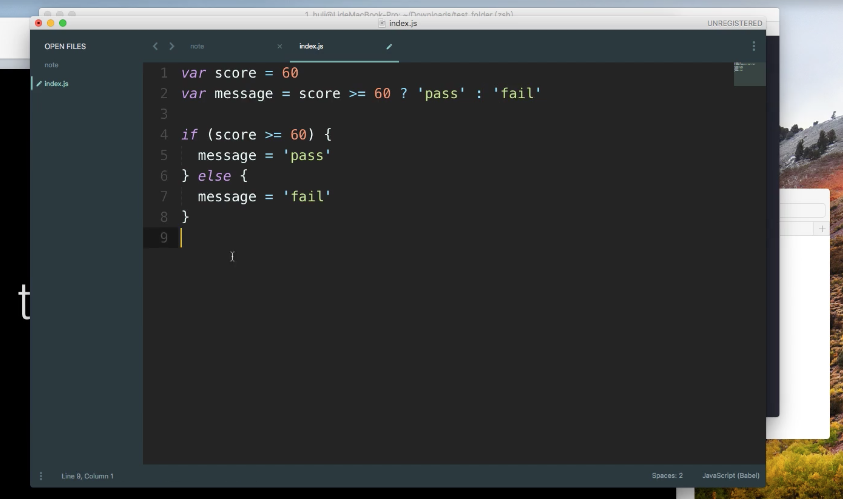score >= 60 ? "pass":"fail" => 判斷條件 ? truefalse

## do...while... 迴圈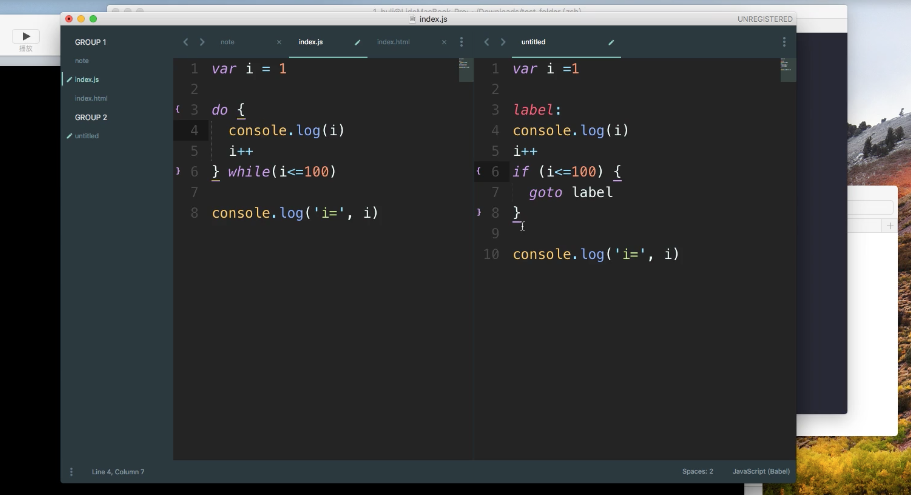break 會終止整個迴圈。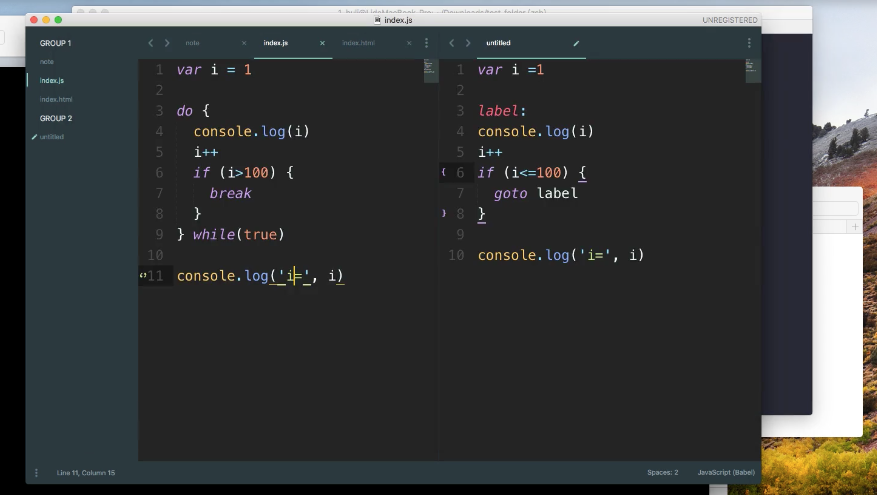continue 可以直接進入下一圈，會跳過後面的指令，如圖所示，如為奇數則會直接進行下一迴圈，不會印出i++ i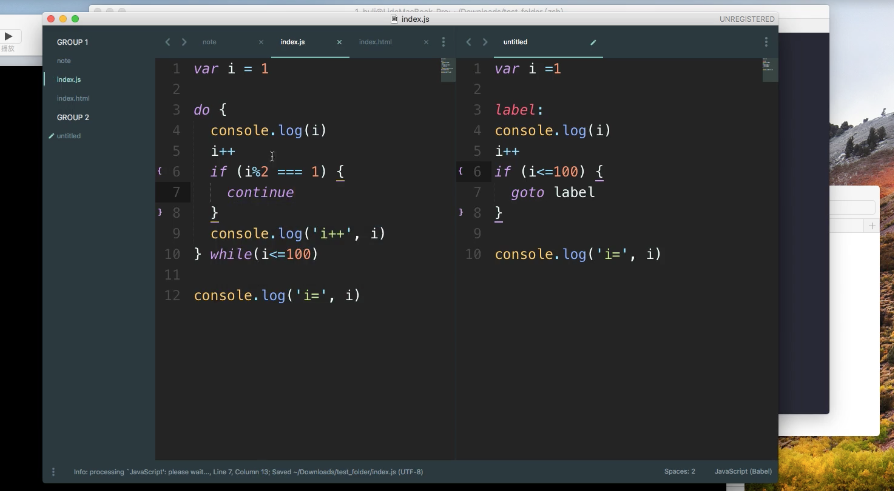## while 迴圈

do...while... 很類似，但反過來，先檢查終止條件，後進行後續動作，大部分情況更常使用 while 迴圈
do...while...while 迴圈 的差異：在條件不成立的情況下，do...while... 還是會跑第一圈。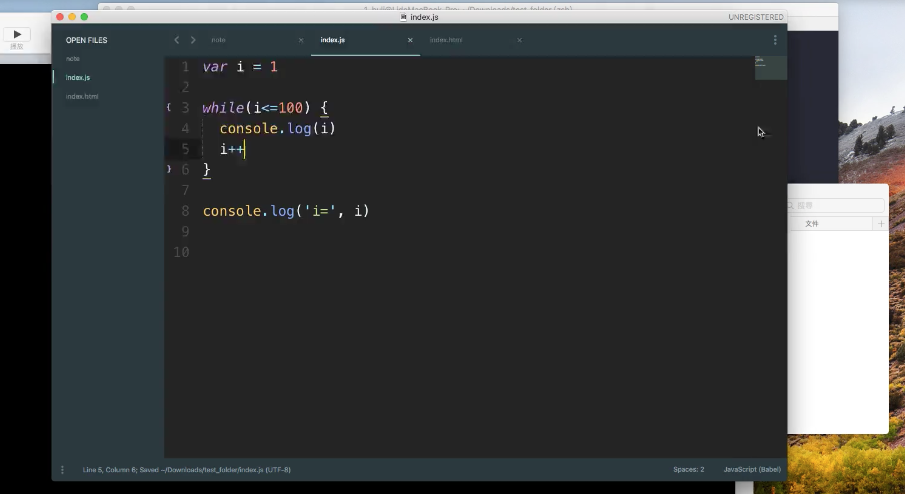## for loop 迴圈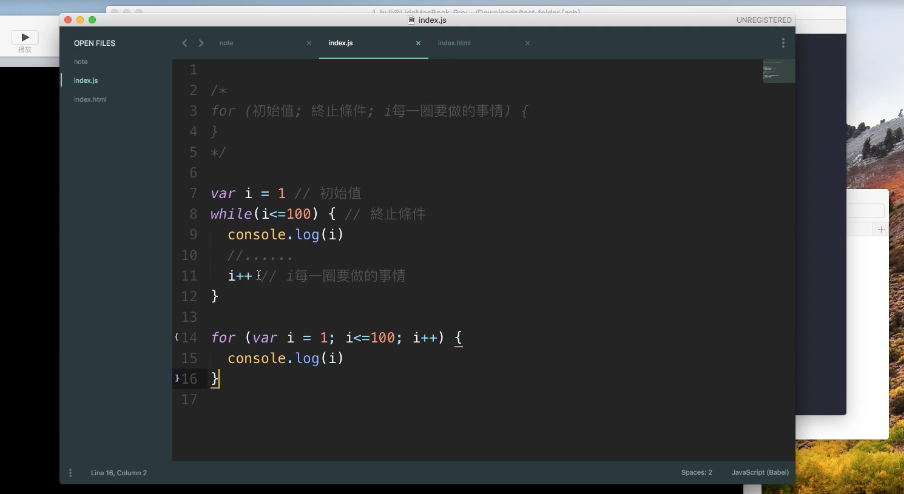## 關於 return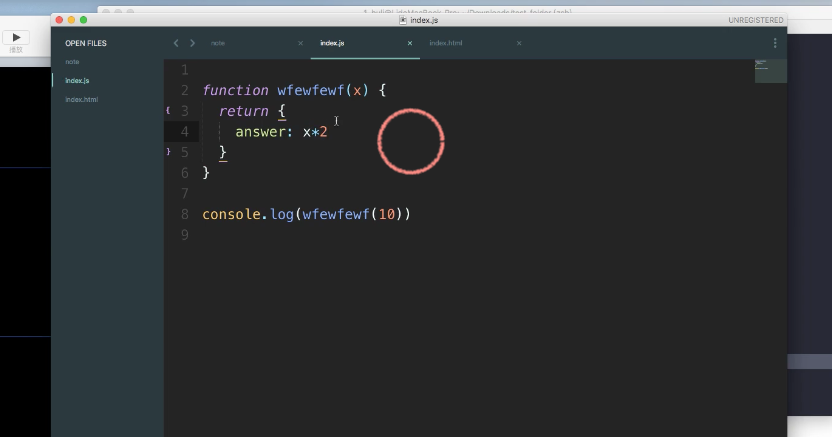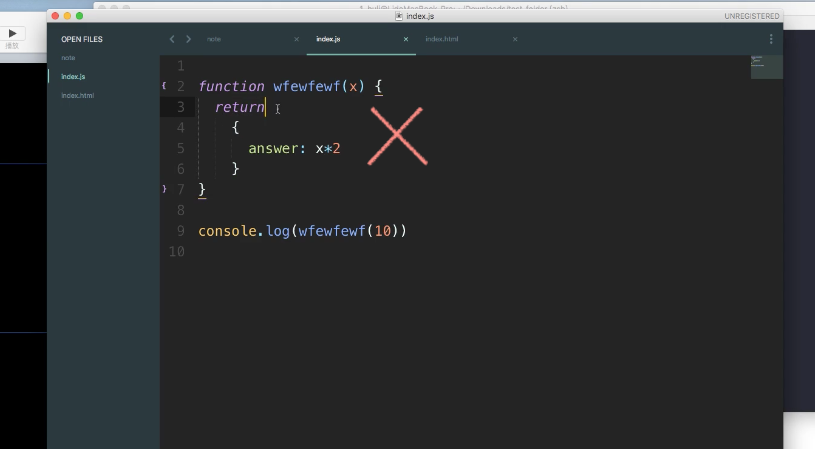## 引數（Arguments）與參數（Parameter）

JavaScript 的內建物件，很類似陣列，用到機率很少，知道即可。

function add (a + b){
return a + b
}
console.log(add(3 + 4))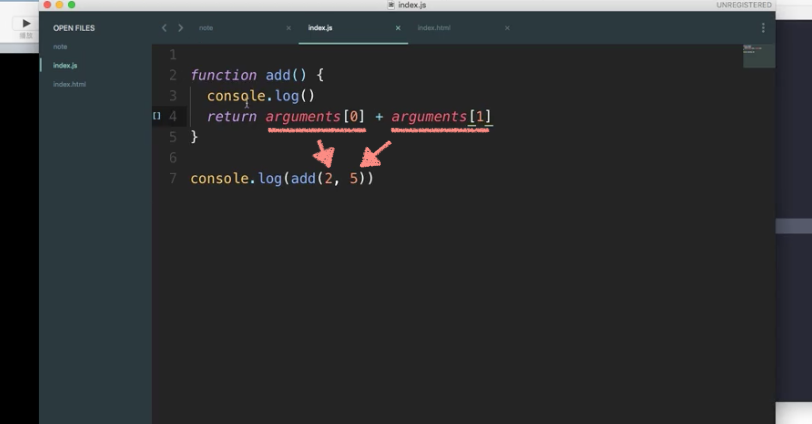## Number 類型的內建函式

parseFloat() 將值轉為數字型態，並保留小數點。

var a = 3.45623

parseFloat(a) =>3.45623
parseFloat(a.toFixed(2)) =>3.45
parseFloat(a.toFixed()) =>3



Math.cell() 無條件進位

Math.floor() 無條件捨去

Math.round() 四捨五入

Math.sqrt() 開根號

Math.pow(x,y) 次方，x 的 y 次方。

Math.random() 產生隨機數，產生的值為 0 ～ 1（包含 0、不包含 1）。

toString() 數字轉字串

var a = 5



Number
Math

## String 類型的內建函式

toUpperCase() 變大寫

toLowerCase() 變小寫

charCodeAt(0) 將位置 0 的 ASCII code 找出。

String.fromeCharCode(65) 將 ASCII code 為 65 的值轉為字串。

indexOf() 尋找關鍵字的位置

var str = "this is a book"

str.indexOf("is") => 5



replace() 取代字串

var str = "this is a book"

str.replace("book","notebook") => str = "this is a notebook"


var str = "this is a book, and I vary like this book."

str.replace("/book/g","notebook") => str = "this is a notebook, and I vary like this notebook."



split() 將字串切開變成陣列

var str = "this is a book"

str.split(" ") => str = ["this", "is", "a", "book"]



trim() 去掉前後空格

length 計算有幾個字

String
ASCII

## Array 類型的內建函式

join() 將陣列用某個元素組合成「字串」

var arr = [1, 2, 3]

arr.join("&") => arr = 1&2&3



map() 可將陣列中的元素都帶入 map() 中的函式進行運算

var arr = [1, 2, 3]

arr.map(double) => arr = [2, 4, 6]



filter() 篩選

var arr = [1, 2, 3]

arr.filter(function(x)){
return x > 1
}

=> [2, 3]



slice() 取某段的值

var arr = [1, 2, 3, 4 ,5, 6]

arr.slice(3) => arr = [4 ,5, 6]
arr.slice(3, 4) => arr = 



splice() 替換陣列的值

var arr = ["a", "b", "c", "d"]

arr.splice(2, 0, "A") => arr = ["a", "b", "A", "c", "d"]
arr.splice(2, 1) => arr = ["a", "b", "d"]
arr.splice(2, 1, "A") => arr = ["a", "b", "A", "d"]

arr.splice(目標位置, 刪除幾個值, 插入/替換的值)


sort() 排序

var arr = [1, 30, 4, 21]

arr.sort() => arr = [1, 21, 30, 4]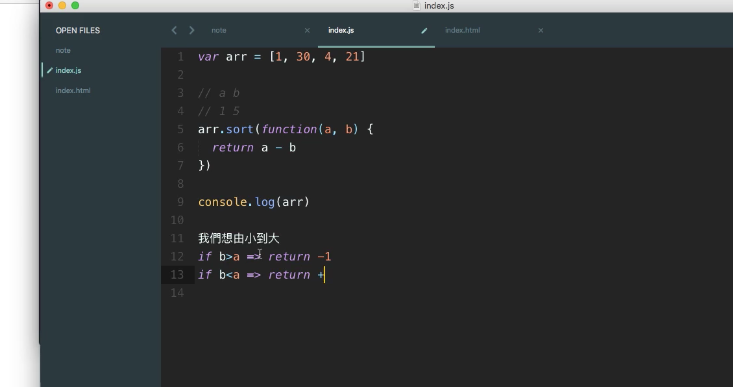2020.6.30

• 依舊花了很長篇幅，但還是沒全數整理完，留下一小部分再來看是更新在這篇還是另開新篇。但終於是告一個段落了，可以快點往後進行其他課程很開心，進度告急一直蠻焦慮的...